## Friday, 7 August 2015

### Driving the VGA Port Using the Elbert V2 FPGA Development Board

I haven't done much with FPGA development for a while...I have been busy with other things. A blog reader has asked me to write a post on using an FPGA to drive a VGA cable. VGA stands for Video Graphics Array. It's a method connected a television monitor to electronic hardware to provide a visual display.

Wikipedia Entry on Video_Graphics_Array

The connector itself is a 15 pin D-type connector which is shown belowWikipedia Entry on VGA Connector

There are is a great deal of information available about the VGA standard and how it works. I did write a considerable amount of information about it but to be honest it has been better written elsewhere. If more information is required on how VGA works then please click the link below. It contains a great deal of data.

A power point presentation on how VGA works

Essentially a VGA signal is a method of controlling a cathode ray tube to display pixels. The pixel is drawn in lines and columns controlled by synchronisation pulses. The resolution of the image is set by the number of lines and columns. For a screen resolution of 640 x 480 there are 640 columns and 480 lines made up of Red, Green and Blue pixels. The display is updated at 60 Hz and this is known as the refresh rate - that means the display is updated every 1/60th of a second or 0.0168 seconds.

From the information in the slides and the paragraph above we can now look to develop a method of generating VGA signals using the Elbert V2 FGPA development board.

Now load up WebISE 14.7 and start a new VHDL project using the appropriate settings for the Elbert V2 development board:I called mine Elbert_VGA but you can use any suitable name that you like - just be aware of where you choose to store the project as we will need to find it later. Click 'Next' to continue.Make sure the above settings are selected to ensure that the correct FPGA device is selected and that VHDL is chosen as the preferred language. Click 'Next' to continue.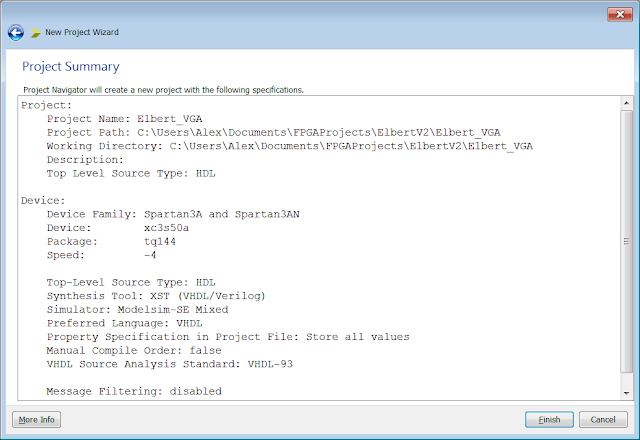The project summary window is displayed. Click finish and then the main project window is displayed: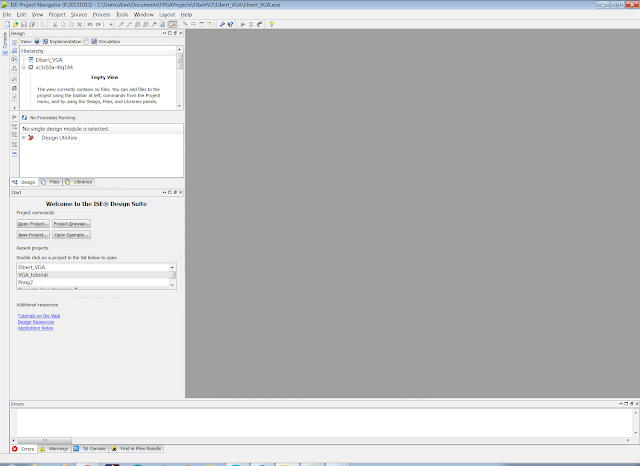Now we need to add some files and start coding. Lets take a step back a moment and discuss what we need to write the code to achieve:

We need to make the FPGA generate the signals required to drive the VGA port. These are:
• Clock
• Reset
• Hsync
• Vsync
• Red
• Blue
• Green
• Row
• Column
The clock we need to generate is 25.17 MHz. We are going to use a special feature of Xilinx WebIse 14.7 to generate the clock. We basically use a wizard feature, tell the wizard the speed of onboard clock of the Elbert V2 development board and then multiply it up to 100 MHz. We then will divide this clock down to 25 MHz as required to synchronize the Hsync and Vsync at 640 x 480 pixels. Most computer monitors will be happy enough to display an image with a 25 MHz clock...strictly speaking it should be 25.17 MHz but who is checking!!

The reset signal will be used to clear the screen. Always a good idea to have a reset!

The Hsync signal is a synchronization pulse that defines how long it takes to draw the pixels in one line. This is known as a scanline and in this case there are 480 pixels in a scanline. The Hsync signal is active low so it moves from 5 Vdc to 0 Vdc in a pulse every 32 µs. Mixed in with the Hsync pulses is something called the front porch and back porch....The Front porch is a signal before the Hsync pulse to clear the screen and lasts for 0.94 µs. The Hsync pulse occurs and then there is another signal known as the back porch which lasts for 1.89 µs. After that there is a 25 µs section which is the time it takes to draw a complete scanline of pixels!

The Vsync signal is a synchonisation pulse that defines how long it takes to draw the 640 scanlines basically how long it takes to draw the entire screen or frame. The Vsync signal is also active low - 5 Vdc to 0 Vdc in a pulse every 16 ms. The Vsync pulse is also preceded by a front porch and back porch signal which last for 0.318 ms and 1.048 ms.

The Red, Green, and Blue signals are simply the signals which define the colour of the pixel to be displayed. They are positive 0.7 Vdc signal that will be generated by the FPGA. A hexadecimal number will be used to select the colour.

The Row and Column signals are really just a method to keep a track of where the current pixel being drawn.

Let's implement this in WebISE 14.7. Right click on the Hierarchy window and click add new source...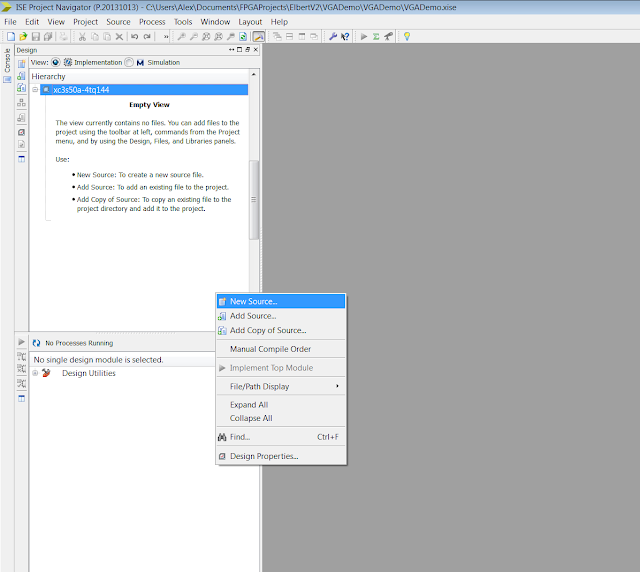Select add vhdl module and give it a suitable name - I called mine VGA_syncClick Next to continue...Add the port names and types as shown in the image below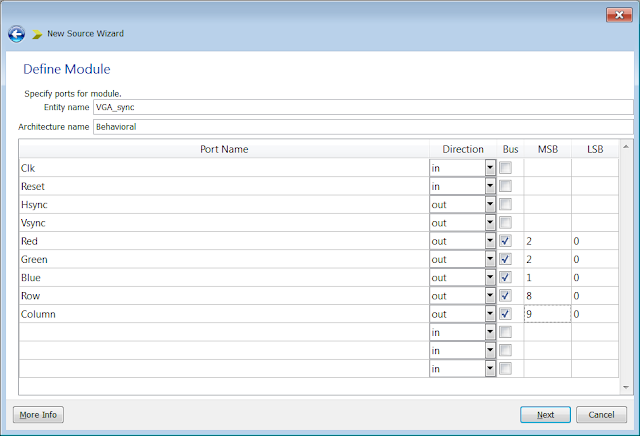Click Next to continue and display the summary window...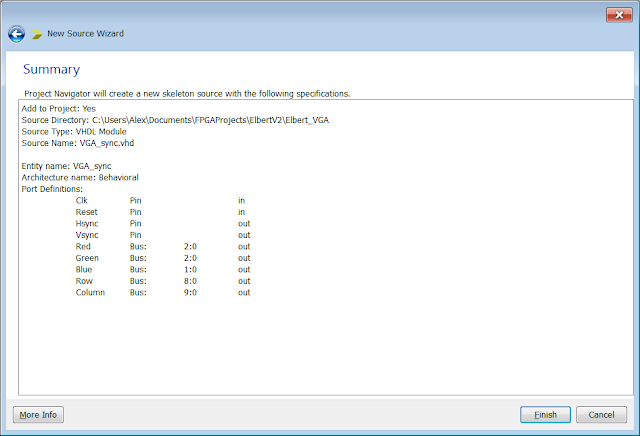Next lets create the clock signal. For this we are going to use an inbuilt wizard feature in WebISE 14.7. We need to generate a 100 MHz clock from the external 12 MHz clock source on the Elbert V2 Development board.

Right click on the hierarchy window again and select add new source...Select IP(CORE Generator and Architecture Wizard). Give it a suitable name - I called mine clocking_inst.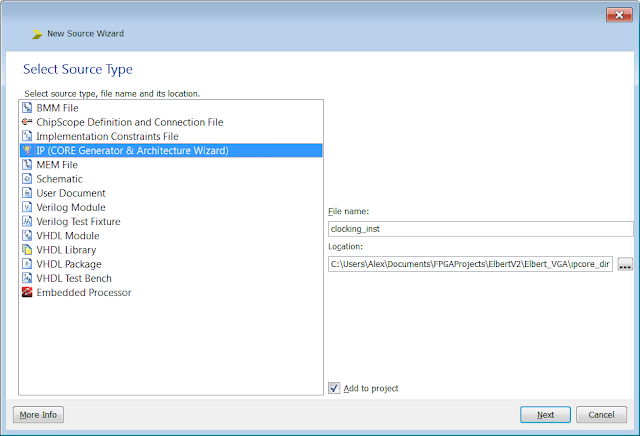click next to continue...And Navigate the FPGA Features and design. Then find Spartan 3E and then Single DCM_SPClick Next to continue...It will take a few moments to generate the core. When ready the screen below will be displayed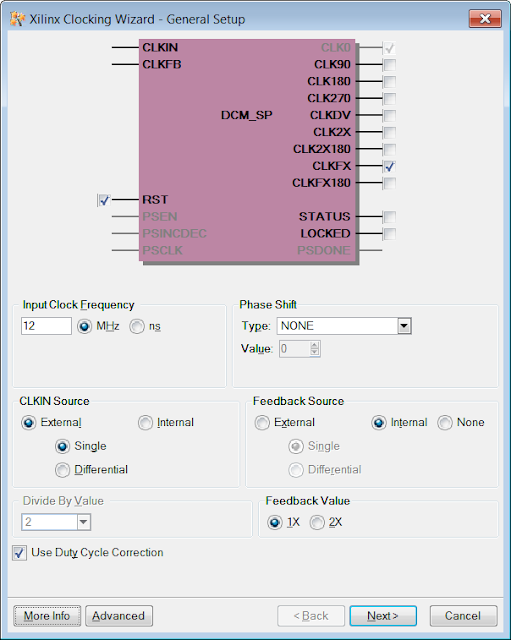Enter the Input Clock Frequency as 12 MHz as this is the speed of the onboard clock on the Elbert V2 Development board, then ensure all of the other details are the same as the image above and then click Next...Select Use Global Buffers and click Next to continue...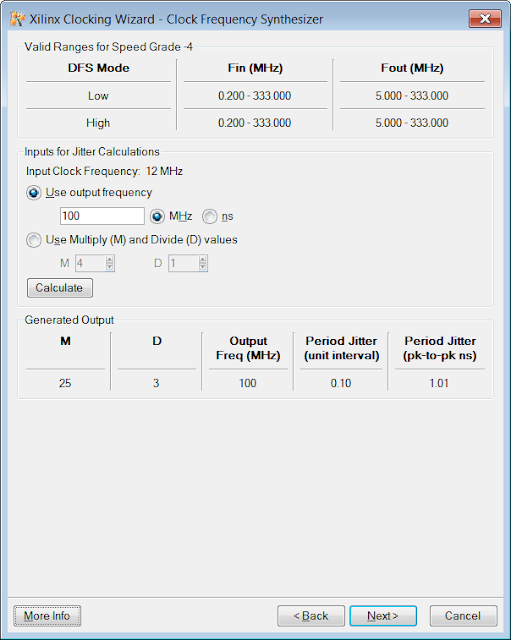Choose to use an output frequency of 100 MHz. We are going to divide this clock down to achieve the 25 MHz clock required to generate the Hsync and Vsync signals for a 640 x 480 frame rate with a 60 Hz refresh rate. Click Next to display the summary page confirming what we have set the wizard to generate.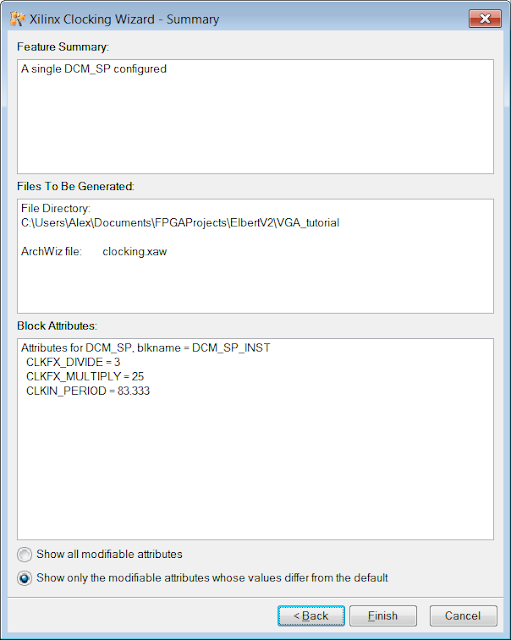Click finish to continue and then the main project screen will be displayed again...Now we have the code written which decides what the pins do and we have automatically generated the code to create a 100 MHz clock. Next we need to write the code which describes how the pins generate the signals...This is the architecture section of the code...

Before we write the code lets look at some pseudo-code which defines what we need to write:

Hcount and Vcount are 10 bit counters

every 1/25,000,000th of a second

if hcount == 799 then
hcount = 0

if vcount == 524 then
vcount = 0

else
vcount = vcount + 1
end if

else
hcount = hcount + 1
end if

if vcount >= 490 and vcount < 492 then
vsync = ’0’
else
vsync = ’1’
end if

if hcount >= 656 and hcount < 752 then
hsync = 0

else
hsync = 1
end if

if hcount < 640 and vcount < 480 then
display a colour on the RGB signals
else
display black colour on the RGB signals
end if

Hold on a minute! Where did all that come from - where did we get the numbers and what does it all that mean?? Lets explain things line by line as much as possible

Hcount and Vcount are 10 bit counters

In order to display a 640 x 480 image at 25.17 MHz we need to have counters to keep track of where the pixels are being drawn in each row and column. Let call them Hcount and Vcount and lets keep them as internal registers....we won't need to every look at them or use them for anything else.

every 1/25,000,000th of a second

A 25 MHz clock changes state 1/25,000,000 times a second. Every time that happens we are going to display the image. Whilst doing that we will ensure that the number of pixels drawn in a line is correct and that the number of columns is correct.

The Hsync timing as mentioned before is 32 µs.

If you multiply the hsync and vsync timings by the 25 MHz clock you will get something close -
to the following numbers:

Horizontal Timings  Duration
(Pixel Clocks)
Visible Area             640
Front Porch              16
Sync Pulse                96
Back Porch               48
Whole line                800
This is because 640 + 16 + 9 + 48 = 800 and therefore this is why we have the pseudo code:

if hcount == 799 then

hcount = 0

It checks if a whole scanline has been displayed and if it has been displayed it resets the hcount to zero to start again.

The vertical timings are as follows...

Vertical Timings   Duration
(Pixel Clocks)

Visible Area         480
Front Porch          10
Sync Pulse            2
Back Porch           33
Whole line            525

This is because 480 + 10 +2 +33 = 525

The code below does the same thing only for the vertical counter:

if vcount == 524 then
vcount = 0

If a whole frame has been displayed reset the vcount.

If a whole frame hasn't been displayed increment the vcount:

else
vcount = vcount + 1
end if

If a whole line hasn't been displayed increment the hcount:

else
hcount = hcount + 1
end if

Now lets discuss the next section of pseudo code:

if vcount >= 490 and vcount < 492 then
vsync = ’0’
else
vsync = ’1’
end if

If the vertical counter is between 490 and 492 do not generate a Vsync pulse because we are in the middle of a Vsync pulse, otherwise generate a Vsync pulse as it is time to display the next frame.

if hcount >= 656 and hcount < 752 then
hsync = 0
else
hsync = 1
end if

If the horizontal counter is between 656 and 752 do not generate a Hsync pulse because we are in the middle of a Hsync pulse, otherwise generate a Hsync pulse as it is time to display the next scanline.

if hcount < 640 and vcount < 480 then
display a colour on the RGB signals

else
display black colour on the RGB signals
end if

If the horizontal counter is less than 640 and the vertical counter is less than 480 then display colours on the screen, if this is not true display nothing (black).

So...now we have explained the pseudo code lets write some VHDL which performs what we want - lets convert the pseudo code to VHDL.

library IEEE;
use IEEE.STD_LOGIC_1164.ALL;
use IEEE.NUMERIC_STD.ALL;
library work;

entity VGA_sync is
Port ( Clk : in  STD_LOGIC;
hsync : out  STD_LOGIC;
vsync : out  STD_LOGIC;
Red : out  STD_LOGIC_VECTOR (2 downto 0);
Green : out  STD_LOGIC_VECTOR (2 downto 0);
Blue : out  STD_LOGIC_VECTOR (2 downto 1)
);
end VGA_sync;

architecture Behavioral of VGA_sync is

-- Intermediate register used internally
signal clock: std_logic := '0';

-- Internal register to store the colour required
signal rgb: std_logic_vector(7 downto 0);

-- Set the resolution of the frame - 640 x 480
signal hCount: integer range 0 to 1023 := 640;
signal vCount: integer range 0 to 1023 := 480;

-- Set the count from where it should start
signal nextHCount: integer range 0 to 1023 := 641;
signal nextVCount: integer range 0 to 1023 := 480;

begin

-- Divide the clock from 100 MHz to 50 MHz
divideClock: process(Clk)
begin
if rising_edge (Clk) then
clock <= not clock;
end if;
end process;

-- create a file which contains the code which causes something to be displayed.
-- The clock which should be given here is 50MHz
VGA_display: entity work.VGA_display
port map(
clock =>  clock,
hcounter  =>  nextHCount,
vcounter => nextVCount,
pixels  => rgb
);

-- The process is carried out for every positive edge of the clock
vgasignal: process(clock)

variable divide_by_2 : std_logic := '0';

begin

-- Make sure the process begins at the correct point between sync pulses
if rising_edge(clock) then

-- Further divide down the clock from 50 MHz to 25 MHz
if divide_by_2 = '1' then

-- Has an entire scanline been displayed?
if(hCount = 799) then
hCount <= 0;

-- Has an entire frame been displayed?
if(vCount = 524) then
vCount <= 0;

else
vCount <= vCount + 1;
end if;

else
hCount <= hCount + 1;
end if;

-- Once the Hcounter has reached the end of the line we reset it to zero
if (nextHCount = 799) then
nextHCount <= 0;

-- Once the frame has been displayed then reset the Vcounter to zero
if (nextVCount = 524) then
nextVCount <= 0;
else
nextVCount <= vCount + 1;
end if;

else
nextHCount <= hCount + 1;
end if;

-- Check if the Vcount is within the minimum and maximum value for the vertical sync signal
if (vCount >= 490 and vCount < 492) then
vsync <= '0';
else
vsync <= '1';
end if;

-- Check if the Hcount is within the minimum and maximum value for the horizontal sync signal
if (hCount >= 656 and hCount < 752) then
hsync <= '0';
else
hsync <= '1';
end if;

-- If the Vcounter and Hcounter are within 640 and 480 then display the pixels.

if (hCount < 640 and vCount < 480) then

--this section of code will cause the display to be Red

Red <= rgb (7 downto 5);
Green <= rgb (4 downto 2);
Blue <= rgb (1 downto 0);
end if;
end if;

-- Set divide_by_2 to zero
divide_by_2 := not divide_by_2;

end if;

end process;
end Behavioral;

So...return to WebISE and the main project window and copy and paste the above code into the architecture section. Save the file. There should not be any errors. Lets explain the code, I'm going to do it section by section...

library IEEE;
use IEEE.STD_LOGIC_1164.ALL;
use IEEE.NUMERIC_STD.ALL;
library work;

entity VGA_sync is
Port ( Clk : in  STD_LOGIC;
hsync : out  STD_LOGIC;
vsync : out  STD_LOGIC;
Red : out  STD_LOGIC_VECTOR (2 downto 0);
Green : out  STD_LOGIC_VECTOR (2 downto 0);
Blue : out  STD_LOGIC_VECTOR (2 downto 1)
);
end VGA_sync;

The first lines above ensure the required library files are included, then the entity statement is written. In VHDL an entity statement define the pins that interact with the actual FPGA device.  In this case we are defining pins which control the clock and the VGA control pins.

architecture Behavioral of VGA_sync is

-- Intermediate register used internally
signal clock: std_logic := '0';

-- Internal register to store the colour required
signal rgb: std_logic_vector(7 downto 0);

-- Set the resolution of the frame - 640 x 480
signal hCount: integer range 0 to 1023 := 640;
signal vCount: integer range 0 to 1023 := 480;

-- Set the count from where it should start
signal nextHCount: integer range 0 to 1023 := 641;
signal nextVCount: integer range 0 to 1023 := 480;

The above lines of code are simply internal variables used to help keep track of where the clock and
the pixels are. Clock is the Clk signal which we divide down. rgb is a register to store which colour
the pixel will be. hCount is an integer variable which counts how many pixels been drawn in a
scanline. vCount is an integer variable which counts how many scanlines have been drawn. The last
two variables nextHCount and nextVCount are present to ensure that once the limits of screen have
been reached that the counters are reset.

begin

-- Divide the clock from 100 MHz to 50 MHz
divideClock: process(Clk)

begin
if rising_edge (Clk) then
clock <= not clock;
end if;
end process;

The above lines of code begin the actual architecture statement. The next lines are a process (kind of
like a function) which divides the 100 MHz clock to 50 MHz. It does this by inverting the Clk signal when that signal is positive. It then copies the state of the Clk to the clock variable which halves the
frequency of the clock.

-- create a file which contains the code which causes something to be displayed.
-- The clock which should be given here is 50MHz

VGA_display: entity work.VGA_display
port map(
clock =>  clock,
hcounter  =>  nextHCount,
vcounter => nextVCount,
pixels  => rgb
);

The above lines of code basically tell the system that there will be another text file which contains the code for actually placing what the designer would like on the display. The parameters which will be
passed to the new file which will be called VGA_display are clock, nextHCount and nextVCount and finally rgb. We will create this file later and write the code required.

-- The process is carried out for every positive edge of the clock
vgasignal: process(clock)

variable divide_by_2 : std_logic := '0';

The above lines of code creates a process (function) and defines a variable called divide_by_2. We
will use this variable to further divide the pixel clock from 50 MHz to 25 MHz.
begin

-- Make sure the process begins at the correct point between sync pulses
if rising_edge(clock) then

-- Further divide down the clock from 50 MHz to 25 MHz
if divide_by_2 = '1' then

The above lines of code tell the device to actually divide the pixel clock from 50 MHz to 25 MHz on the rising edge of the 50 MHz clock.

-- Has an entire scanline been displayed?
if(hCount = 799) then
hCount <= 0;

-- Has an entire frame been displayed?
if(vCount = 524) then
vCount <= 0;

else
vCount <= vCount + 1;
end if;

else
hCount <= hCount + 1;
end if;

The above lines of code check if an entire scanline has been drawn and resets the hCount to zero if this is the case. The same check is performed on the vCount to see if an entire frame has been drawn. If it has then the vCount is reset. If neither hCount or vCount has reached it's limit they are
incremented and the process continues.

-- Check if the Vcount is within the minimum and maximum value for the vertical sync signal
if (vCount >= 490 and vCount < 492) then
vsync <= '0';
else
vsync <= '1';
end if;

-- Check if the Hcount is within the minimum and maximum value for the horizontal sync signal
if (hCount >= 656 and hCount < 752) then
hsync <= '0';
else
hsync <= '1';
end if;

The above lines of code check if the hCount variable has reached the limit for the display resolution.
If it has the count is restarted. The same check is performed on the vCount and if so then the vCount
is restarted.

-- If the Vcounter and Hcounter are within 640 and 480 then display the pixels.

if (hCount < 640 and vCount < 480) then

--this section of code will cause the display to be Red

Red <= rgb (7 downto 5);
Green <= rgb (4 downto 2);
Blue <= rgb (1 downto 0);
end if;
end if;

-- Set divide_by_2 to zero
divide_by_2 := not divide_by_2;

end if;

end process;
end Behavioral;

The above lines of code invert the clock divider and then close off the open if statements and end the process.

Now if things are correct and things have been copied or typed in correctly you should see the
following image in WebISE 14.7. The exclamation mark next to the filename is a warning by the
xilinx software to say that the code is looking for a file - don't worry we haven't created this file yet.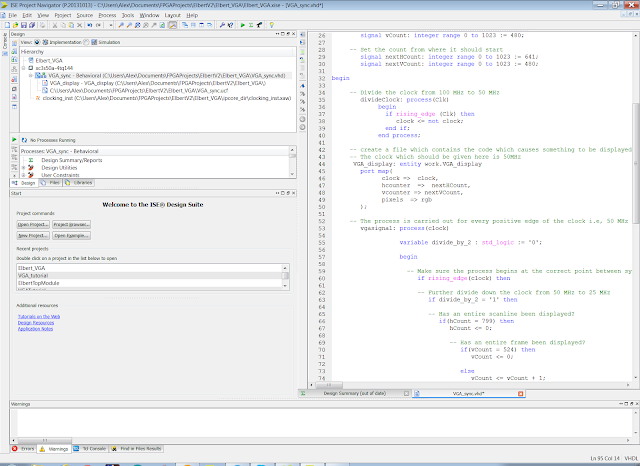Right click on the hierarchy window and select 'add source' - choose VHDL module and call it VGA_display!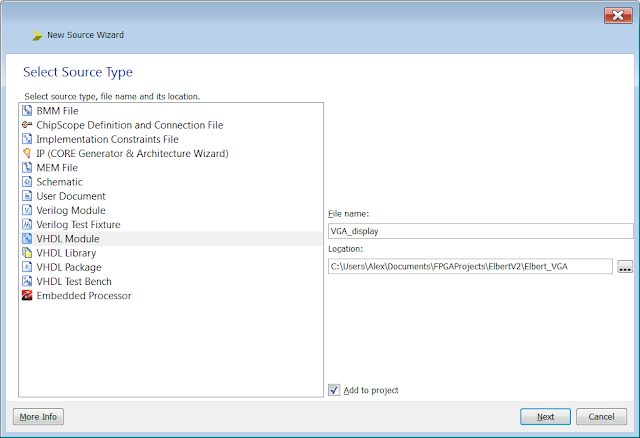Click 'Next' to continue. Don't worry about adding any inputs and outputs we will add them ourselves when we write the code.Click 'Next' to continue and display the summary window...Click finish to return to the main project window and the newly created file:Delete all of the text in the file - we are going to write our own code from scratch. The code has to
basically pass the information of what we want the display to do back to the VGA_sync file. The
information from the VGA_sync file will be passed back to a top module file which will pass the
information to the pins which will drive the VGA port...

For now what we need to do is choose what we want to display on the screen. To keep things simple
for now lets just fill the whole screen with a colour. We can add shapes and other stuff later.

library IEEE;
use IEEE.STD_LOGIC_1164.ALL;
use IEEE.NUMERIC_STD.ALL;

entity VGA_display is port

-- Assuming 50MHz clock.If the clock is reduced then it might give the unexpected output.
clock: in std_logic;

-- The counter tells whether the correct position on the screen is reached where the data is to be displayed.
hcounter: in integer range 0 to 1023;
vcounter: in integer range 0 to 1023;

-- Output the colour that should appear on the screen.
pixels : out std_logic_vector(7 downto 0)
); end VGA_display;

architecture Behavioral of VGA_display is
-- Intermediate register telling the exact position on display on screen.
signal x : integer range 0 to 1023 := 100;
signal y : integer range 0 to 1023 := 80;

begin

-- On every positive edge of the clock counter condition is checked,
video_output: process(clock)
begin
if rising_edge (clock) then
-- If the counter satisfy the condition, then output the colour that should appear.
if (hcounter >= 1) and (hcounter < 480) and (vcounter >= 1) and (vcounter < 640 )
then pixels <= x"F0";
-- If the condition is not satisfied then the output colour will be black.
else
pixels <= x"00";
end if;
end if;
end process;
end Behavioral;

Copy and paste the code above into the VGA_display file and save it. The first section of the code (shown below) is the entity statement which as before defines how the I/O in the code will interact with the other code or with the real world. In this case we are passing data back to the VGA_sync code.

entity VGA_display is port

-- Assuming 50MHz clock.If the clock is reduced then it might give the unexpected output.
clock: in std_logic;

-- The counter tells whether the correct position on the screen is reached where the data is to
-- be displayed.

hcounter: in integer range 0 to 1023;
vcounter: in integer range 0 to 1023;

-- Output the colour that should appear on the screen.
pixels : out std_logic_vector(7 downto 0)

); end VGA_display;

The architecture section of the code shown below defines how the display is going to behave. In this case it will fill the screen with a single colour. We will change it later to do other things...for now lets just get something on the screen.

architecture Behavioral of VGA_display is
-- Intermediate register telling the exact position on display on screen.

signal x : integer range 0 to 1023 := 100;
signal y : integer range 0 to 1023 := 80;

begin
-- On every positive edge of the clock counter condition is checked,

video_output: process(clock)
begin
if rising_edge (clock) then
-- If the counters satisfy the condition, then output the colour that should appear.
if (hcounter >= 1) and (hcounter < 480) and (vcounter >= 1) and (vcounter < 640 )
then pixels <= x"F0";
-- If the condition is not satisfied then the output colour will be black. else pixels <= x"00";
end if;
end if;

end process;

end Behavioral;

Now we need to create another new VHDL module - this will be the project top module - rightclick on the hierarchy window again and add another new VHDL Module - call it something sensible - I
called mine VGA_top_module...Click 'Next' to continue...The define module window will be displayed - there is no need to add anything as again we will define the pins when we write the code.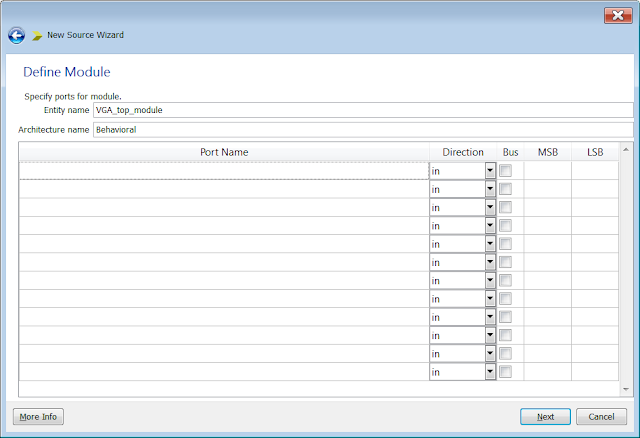Click 'Next' to display the summary window...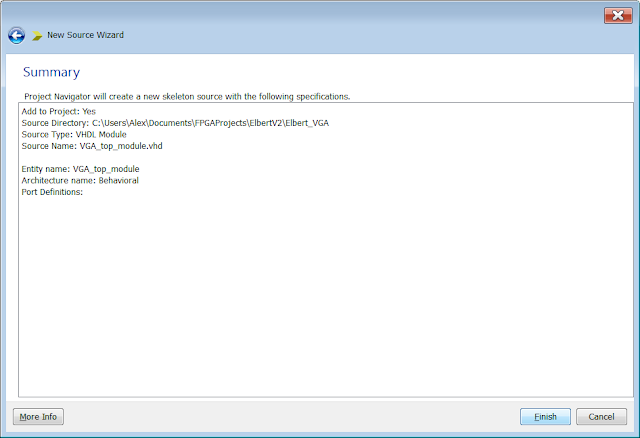Click 'Finish' to return to the main project screen and the newly created file will be openin the text
editor.Delete all of the automatically generated code - we are going to add our own:

library IEEE;
use IEEE.STD_LOGIC_1164.ALL;
use IEEE.NUMERIC_STD.ALL;

entity VGA_top_module is
port (
-- Inputs
-- the only input is the 12MHz input clock...
-- We can add more later!
Clk : IN STD_LOGIC;

-- Output
-- ElbertV2 VGA Display
hsync : OUT STD_LOGIC;
vsync : OUT STD_LOGIC;
Red   : OUT STD_LOGIC_VECTOR(2 downto 0);
Green : OUT STD_LOGIC_VECTOR(2 downto 0);
Blue  : OUT STD_LOGIC_VECTOR(2 downto 1)
);

end VGA_top_module;

architecture Behavioral of VGA_top_module is

component clocking
port(-- Input clock of 12MHz
CLKIN_IN         : in     std_logic;
-- Output clock has to been set to 100 MHz. If input clock frequency
-- changes then update clocking_inst to reflect the change
CLKFX_OUT          : out    std_logic
);
end component;

component VGA_sync
port    (
-- Input Clock 100MHz
Clk   : in std_logic;
-- Output for the VGA Display
hsync    : out std_logic;
vsync    : out std_logic;
Red      : out std_logic_vector(2 downto 0);
Green    : out std_logic_vector(2 downto 0);
Blue     : out std_logic_vector(2 downto 1)
);
end component;

signal   clock   : std_logic := '0';

begin

clocking_inst : clocking
port map (
CLKIN_IN        => Clk,
CLKFX_OUT       => clock
);

VGA_inst : VGA_sync
port map (
Clk   => clock,
hsync => hsync,
vsync => vsync,
Red   => Red,
Green => Green,
Blue  => Blue
);

end Behavioral;

Now to explain the above code....The entity section defines the pins that will actually drive the VGA connector. The Clk pin is the 100 MHz clock. This will be divided down to 25 MHz by the code in in VGA_sync. The other CLK pins are the actual input clock at 12 MHz which >is multiplied up to 100 MHz by clocking_inst. The rest of the pins are the output pins used to drive the VGA connector.The architecture section calls the clocking component and the VGA_sync components. These are the files which contain the code for generating the 100 MHz clock and the VGA_sync code which drives the VGA port. After that the objects are instantiated - a fancy way of saying loaded....

Woohoo...coding complete....Well apart from one small step!

Now before we can actually upload this code to the FPGA device on the Elbert V2 development board we need to tell WebISE 14.7 which pins to use. We do this by adding a user constraints file to the project which defines which tracks connect the VGA connector to the FPGA device pins. We can find this information from the schematic diagram for the Elbert V2 Development board.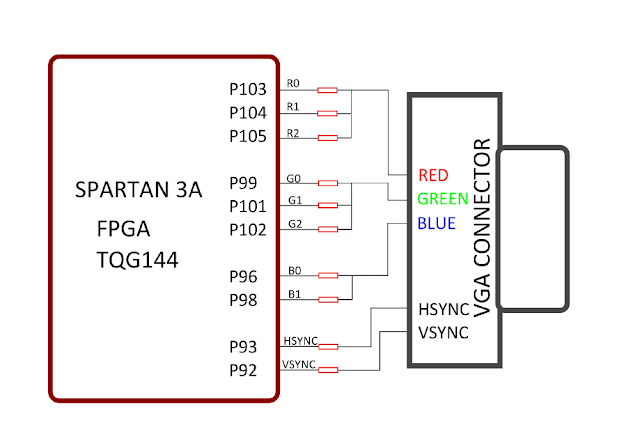From the above information we now know that the red signals are connected to P103, P104
and P105. The blue signal is connected to P96 and P98 and the green signal is connected to P99,
P101 and P102.

Finally hsync and vsync are connected to P93 and P92. We also know that the external clock to the FPGA device is 12 MHz and the voltage supply is 3.3 Vdc. This is all the information required to writethe implementation constraints file.

In the Main project window in webISE 14.7 right click on the hierarchy window and add 'new source'The following window will be displayed. Select 'Implementation Constraints file' and give it a suitable file name...Click next to continue...Click Finish to return to the main project window with the new file open. Copy and paste the code below into the file:

#*****************************************#
#   UCF for ElbertV2 Development Board    #
#*****************************************#
CONFIG VCCAUX = "3.3" ;

# Clock 12 MHz
NET "Clk" LOC = P129  | IOSTANDARD = LVCMOS33 | PERIOD = 12MHz;

############
# VGA    #
############

NET "hsync"              LOC = P93  | IOSTANDARD = LVCMOS33 | SLEW = SLOW | DRIVE = 12;
NET "vsync"              LOC = P92  | IOSTANDARD = LVCMOS33 | SLEW = SLOW | DRIVE = 12;

NET "Blue"            LOC = P98  | IOSTANDARD = LVCMOS33 | SLEW = SLOW | DRIVE = 12;
NET "Blue"            LOC = P96  | IOSTANDARD = LVCMOS33 | SLEW = SLOW | DRIVE = 12;

NET "Green"           LOC = P102  | IOSTANDARD = LVCMOS33 | SLEW = SLOW | DRIVE = 12;
NET "Green"           LOC = P101  | IOSTANDARD = LVCMOS33 | SLEW = SLOW | DRIVE = 12;
NET "Green"           LOC = P99   | IOSTANDARD = LVCMOS33 | SLEW = SLOW | DRIVE = 12;

NET "Red"             LOC = P105  | IOSTANDARD = LVCMOS33 | SLEW = SLOW | DRIVE = 12;
NET "Red"             LOC = P104  | IOSTANDARD = LVCMOS33 | SLEW = SLOW | DRIVE = 12;
NET "Red"             LOC = P103  | IOSTANDARD = LVCMOS33 | SLEW = SLOW | DRIVE = 12;

Save the project and then you will need to set the top module as the top module. To be honest while documenting how to do all of this I created the files in the incorrect order. There is away of fixing this within Xilinx ISE but I'm not sure how to do it. I repeated all of the above steps and created the files in the correct order. I have provided a link to the project files here which are correct.

Elbert_VGA project files

Download the project, extract it to a directory of your choice and then reload the project in Xilinx WebISE 14.7 - My Apologies about this I started with the VGA_sync implementation first which was a mistake. In future I'll discuss creating the top module first (experience...)

It's finally time to 'implement a top level module'. Click on the green arrow and WebISE 14.7 will convert all of the code required ready to create the binary configuration file. Now create a binary configuration file and upload it to the Elbert V2 Development board.

If all went according to plan this file can now be uploaded the the development Go ahead load up the Elbert V2 Configuration tool....You will need to select the COM port that the development board is connected to. You will also need to navigate to the directoryfolder where the project is stored and then select the newly written BIN file - it will be called VGA_top_module.bin - Program the device!!!

Once you have programmed the device the following image should be displayed. If you made it this far - WELL Done!

Here is what should be present on the monitor - connect up your FPGA development board to a VGA monitor and see if it looks the same!

If you want to change the colour or reduce the size of the box then you need to edit the VHDL code in the VGA_display.vhd file.  The hex value assigned to pixels changes the colour where FF is white and 00 is black - there are 64 different colours available.  The size of the box is modified by changing the values for hcounter and vcounter in the if statement....

if (hcounter >= 1)  and (hcounter < 480) and (vcounter >= 1) and        (vcounter < 640
) then pixels <= x"F0";

Here is a short video of my board working - I changed the code slightly to display a small 100 x 100 white box!

That's all people - I will try and post some more FPGA tutorials soon.

Good luck - Langster!